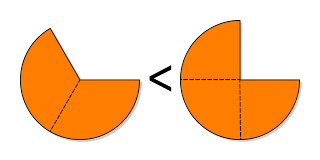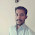# Mental Maths (Comparison of Fractions) - Part 1

You have to Compare the given fractions in a number of problems in Data Interpretation and Quantitative Ability. Let us study some of the common methods of identifying out the largest or smallest of a given set of fractions which are useful for Mental Maths.

### Model 1 :

When the numerators are same and the denominators are different, the fraction with the largest denominator is the smallest. Have a look at the following example.
• Which of the following fractions is the smallest?
• (3/5) , (3/7) , (3/13), (3/8)• Here, 13 is the largest denominator, so, (3/13) is the smallest fraction. 5 is the smallest denominator, hence (3/5) is the largest fraction.
• Here logic is very simple,
• Situation 1 : Assume that you are 5 Children in your family. Your Dad brought an Apple and mom cut it into 5 pieces and distributed among all the children including you. (1/5)
• Situation 2 : Assume that you are 8 Children in your family. Your Dad brought an Apple and mom cut it into 8 pieces and distributed among all the children including you. (1/8)
• In which Situation will you get the BIG Piece of the Apple??? ;). Obviously in the Situation 1. Thats it.........

### Model 2 :

When the numerators are different and the denominators are same, the fraction with the largest numerator is the largest. Have a look at the following example.
• Which of the following fractions is the smallest?
• (7/5) , (9/5), (4/5), (11/5)
• As 4 is the smallest numerator, the fraction 4/5 is the smallest.
• As 11 is the largest numerator, the fraction 11/5 is the largest.
• Here too logic is very simple,
• Situation 1 : Assume that you are 4 Children in your family. Your Dad brought 8 Apples and mom distributed them among all the children including you. (8/4)
• Situation 2 :  Assume that you are 4 Children in your family. Your Dad brought 12 Apples and mom distributed them among all the children including you. (12/4)
• In which situation will you get more apples? Obviously in the second. So, 12/4 is the Biggest.. Thats it ;)

### Model 3 :

The fraction with the largest numerator and the smallest denominator is the largest.
• Which of the following fractions is the largest?
• (19/16), (24/11), (17/13), (21/14), (23/15)
• As 24 is the largest numerator and 11 is the smallest denominator, 24/11 is the largest fraction.
• Logic??? Cutting the More fruits into Less pieces is more beneficial for you than cutting the Less fruits into More pieces :P

### Model 4 :

When the numerators of two fractions are unequal, we try and equate them by suitably cancelling factors or by suitably multilplying the numerators. Thereafter we compare the denominators as in Model 1. Have a look at the following examples.
• Which of  the following fractions is the largest?
• (64/328), (28/152), (36/176), (49/196)
• 64/328 = 32/164 = 16/82 = 8/41 this is approximately equal to 1/5
• Note : In these type of problems, approximate values will be enough. No need to get EXACT values.
• 25/152 = 14/76 = 7/38 this is approximately equal to 1/5.5
• 36/176 = 18/88 = 9/44 this is approximately equal to 1/5
• 49/196 = 7/28 = 1/4
• As all the numerators are 1 and the least denominator is 4, the fraction 49/196 is the largest
• Which of the following fractions is the largest?
• (71/181), (214/519), (429/1141)
• (71/181) = (71 X 6) / (181 X 6) = 426/1086
• (214/519) = (214 X 2) / (519 X 2) = 428/1038
• The numerators are now all ALMOST equal (426, 428 and 429). The smallest denominator is 1038.
• So, the largest fraction must be 428/1038  that is 214/519 :)

1.I was just browsing through the page & I must appreciate whoever did the logic & wrote the situation analysis here... Good job! Was a refreshing read, thanks! Quite innovative...

1.2.wow it was very nice,mam Iam non maths student till know Iam very afraid about these types of problems now Iam fan to solve.thanku............

3.Simply superb...........

4.superb...............

5.6.thank u very much

7.i hate math..but by seeing funda like these i m falling in love wid math...!!!!! thanks admin....gr8 job

8.good article,really helped me.Fractions were troubling me a lot.

9.10.Its awesome di.... nice job ....

11.i appreciate the person who did write the situation analysis here. its a best site forever for banking aspirants thank u very very very much. its such a useful site.

12.very good concept

13.fantastic Gr8 ambunz.. you are notes are awesome
thank u so much

14.very nice concept...compltely clear in mind....thank u soooo much....

15.great website......... Thank you so much.

16.17.hyy this is really hlpful .. grt website.. thnks a lot 4 ur wndrful wrk. n giving me such a useful material

18.I always skipped questions from fractions (if not same numerator or denominator).. this article seems really helpful .. thank you again gr8ambitionz ... cheers

19.I want to appreciate gr8ambitionz Team for there excellent work.

20.Very well conceptual wise making the aspirants to understand

21.22.its very easy to undustand very very nice thank u for giving these type suggustions to the students thank u

23.superbbbb i love maths from this we can improve our knowledge more please update new logics daily

24.25.superbbbb nice class

26.very well done just superb :)

27.Awesome
Got it well as never before

28.An excellent article. Explanation is very simple

29.What a creative explanation ...thanks to whole gr8ambitionz TEAM

30.madam thank u so much for taking risk in providing these materials,madam i would like to know how u done the logic for the last example by multiplying with 6 & 2?can u pls explain it?

31.madam explain the model 4 last part Q:71/181 i didnt understand?

32.really good one,keep it up and thnq gr8ambitionz...................................
u r helping us a lot..................

33.Gr8 Now i started loving maths

34.hi really awesome, nice social service. but i want pdf files for all

35.Excellent Site...Never found such informative material online

36.solving such problems without pen and paper and with good speed would obviously increase confidence

37.solving such problems with good speed and without approach of pen and paper will obviously increase confidence

38.thank u so much

39.thank you so much..

40.in model 3 which one would be the second largest???

41.what logic can be used for this quest:: 2/7 ,8/9 ,4/5??

42.What logic will be used in this query::: 6/7, 8/9, 4/5??

1.Its similiar to Model 4 ..
6/7 8/9 4/5
break it down
= 1/1.16 1/1.125 1/1.25
here 1.125 is smallest
therefore, 8/9 is largest fraction and 4/5 is least.
Simple .

43.really like this work .please keep it continue...

44.you are genius mam ...awesome

45.its very helpful.. but I need practice on it.. Confused when to use which methood

1.APPLY YR MIND

46.47.48.